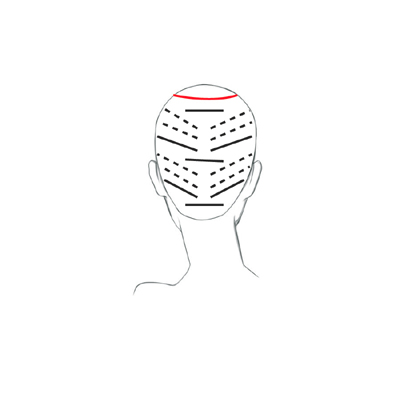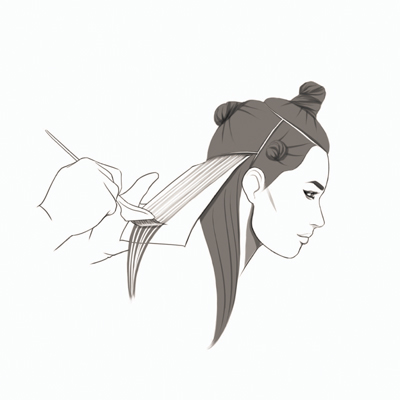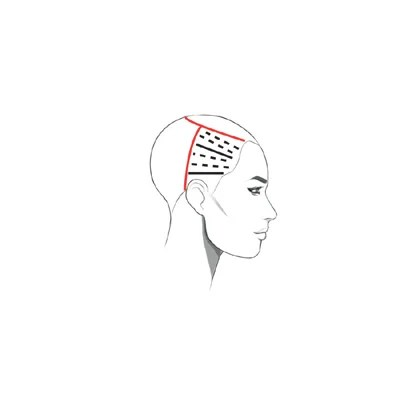Sandalwood Swirl
Last updated: May 30, 2017

# Sandalwood Swirl

ALL PHOTOS SLIDESHOW
GET THE HOW-TO• Natural Level

8

• Formula A

Matrix SOCOLOR 7CG + 10-volume Matrix Cream Developer

• Formula B

Matrix Light Master + 10-volume Cream Developer

• Formula C

Matrix Color Sync 8CG + 10-volume Cream Developer

• Formula D

equal parts Color Sync 10CG +10G + 10-volume Cream Developer# Sandalwood Swirl

Inspired by the unique mixture of highs and lows found in light wooden panels, this shade from the Matrix Designer Woods Collection strikes the perfect balance for blonde clients looking for soft depth and warmth. Wearable copper reflects with hints of gold for gorgeous, natural vibrancy.

Manufacturer: Matrix, @matrix

## COLOR FORMULAS• Natural Level

8

• Formula A

Matrix SOCOLOR 7CG + 10-volume Matrix Cream Developer

• Formula B

Matrix Light Master + 10-volume Cream Developer

• Formula C

Matrix Color Sync 8CG + 10-volume Cream Developer

• Formula D

equal parts Color Sync 10CG +10G + 10-volume Cream Developer

## HOW-TO STEPS•• Natural Level

8

• Formula A

Matrix SOCOLOR 7CG + 10-volume Matrix Cream Developer

• Formula B

Matrix Light Master + 10-volume Cream Developer

• Formula C

Matrix Color Sync 8CG + 10-volume Cream Developer

• Formula D

equal parts Color Sync 10CG +10G + 10-volume Cream Developer

1

Create a horseshoe shaped section on the top of the head—this is Section 4. Create a parting from behind the ear to the top of the parietal on both sides and secure—these are Sections 2 and 3. The entire back is Section 1. Subdivide for control.

In Section 1, above the hairline, create a fine center slice. Apply Formula A from scalp to ends.

•• Natural Level

8

• Formula A

Matrix SOCOLOR 7CG + 10-volume Matrix Cream Developer

• Formula B

Matrix Light Master + 10-volume Cream Developer

• Formula C

Matrix Color Sync 8CG + 10-volume Cream Developer

• Formula D

equal parts Color Sync 10CG +10G + 10-volume Cream Developer

2

Skip a half-inch section. Towards the right, create a diagonal slice. Apply Formula A scalp to ends. Leave a small space and create a diagonal weave. Apply Formula B. Create another diagonal weave directly above, again using Formula B.

•• Natural Level

8

• Formula A

Matrix SOCOLOR 7CG + 10-volume Matrix Cream Developer

• Formula B

Matrix Light Master + 10-volume Cream Developer

• Formula C

Matrix Color Sync 8CG + 10-volume Cream Developer

• Formula D

equal parts Color Sync 10CG +10G + 10-volume Cream Developer

3

On the opposite side, continue working by placing a diagonal slice and two weaves. Continue working in the same manner, using the same pattern, beginning with a center slice. Complete Section 1.

•• Natural Level

8

• Formula A

Matrix SOCOLOR 7CG + 10-volume Matrix Cream Developer

• Formula B

Matrix Light Master + 10-volume Cream Developer

• Formula C

Matrix Color Sync 8CG + 10-volume Cream Developer

• Formula D

equal parts Color Sync 10CG +10G + 10-volume Cream Developer

4

Release side Section 2. At the bottom of the section create a diagonal slice from the temporal region. Apply Formula A scalp to ends.

•• Natural Level

8

• Formula A

Matrix SOCOLOR 7CG + 10-volume Matrix Cream Developer

• Formula B

Matrix Light Master + 10-volume Cream Developer

• Formula C

Matrix Color Sync 8CG + 10-volume Cream Developer

• Formula D

equal parts Color Sync 10CG +10G + 10-volume Cream Developer

5

Leave a small space and create a diagonal weave beginning just behind the temporal region. Foils will begin pivoting and staggering. Apply Formula B. Create another diagonal weave and apply Formula B. (Tip: Slightly staggering the foils at the temporal region will help you to avoid a bright spot of color.) Continue working in the same manner, placing a slice and two weaves, all pivoting from the temporal region. Repeat in Section 3.

•• Natural Level

8

• Formula A

Matrix SOCOLOR 7CG + 10-volume Matrix Cream Developer

• Formula B

Matrix Light Master + 10-volume Cream Developer

• Formula C

Matrix Color Sync 8CG + 10-volume Cream Developer

• Formula D

equal parts Color Sync 10CG +10G + 10-volume Cream Developer

6

In Section 4, begin above the right eye. Continue using the same pattern of diagonal slicing using Formula A followed by two diagonal weaves using Formula B.

•• Natural Level

8

• Formula A

Matrix SOCOLOR 7CG + 10-volume Matrix Cream Developer

• Formula B

Matrix Light Master + 10-volume Cream Developer

• Formula C

Matrix Color Sync 8CG + 10-volume Cream Developer

• Formula D

equal parts Color Sync 10CG +10G + 10-volume Cream Developer

7

Apply Formula C to all remaining hair. Process, rinse, shampoo and rough dry. Section the head into quadrants. Apply Formula D scalp to ends. Process, rinse, shampoo, condition, dry and style.

•• Natural Level

8

• Formula A

Matrix SOCOLOR 7CG + 10-volume Matrix Cream Developer

• Formula B

Matrix Light Master + 10-volume Cream Developer

• Formula C

Matrix Color Sync 8CG + 10-volume Cream Developer

• Formula D

equal parts Color Sync 10CG +10G + 10-volume Cream Developer

8

Finished Look